## Introducing “ei-Recall” – A New Gold Standard for Recall Calculations in Legal Search – Part ThreePlease read Part One and Part Two of this article before reading this third and final segment.

First Example of How to Calculate Recall Using the ei-Recall Method

Let us begin with the same simple hypothetical used in In Legal Search Exact Recall Can Never Be Known. Here we assume a review project of 100,000 documents. By the end of the search and review, when we could no longer find any more relevant documents, we decided to stop and run our ei-Recall quality assurance test. We had by then found and verified 8,000 relevant documents, the True Positives. That left 92,000 documents presumed irrelevant that would not be produced, the Negatives.

As a side note, the decision to stop may be somewhat informed by running estimates of possible recall range attained based on early prevalence assumptions from a sample of all documents at or near the beginning of the project. The prevalence based recall range estimate would not, however, be the sole driver of the decision to stop and test. The prevalence based recall estimates alone can be very unreliable as shown In Legal Search Exact Recall Can Never Be Known. That is one of the main reasons for developing the ei-Recall alternative. I explained the thinking behind the decision to stop in Visualizing Data in a Predictive Coding Project – Part Three.

I will not have stopped the review in most projects (proportionality constraints aside), unless I was confident that I had already found all of those (highly relevant) types of documents; already found all types of strong relevant documents, and already found all highly relevant document, even if they are cumulative. I want to find each and every instance of all hot (highly relevant) documents that exists in the entire collection. I will only stop (proportionality constraints aside) when I think the only relevant documents I have not recalled are of an unimportant, cumulative type; the merely relevant. The truth is, most documents found in e-discovery are of this type; they are merely relevant, and of little to no use to anybody except to find the strong relevant, new types of relevant evidence, or highly relevant evidence.

Back to our hypothetical. We take a sample of 1,534 (95%+/-2.5%) documents, creating a 95% confidence level and 2.5% confidence interval, from the 92,000 Negatives. This allows us to estimate how many relevant documents had been missed, the False Negatives.

Assume we found only 5 False Negatives. Conversely, we found that 1,529 of the documents picked at random from the Negatives were in fact irrelevant as expected. They were True Negatives.

The percentage of False Negatives in this sample was thus a low 0.33% (5/1534). Using the Normal, but wrong, Gaussian confidence interval the projected total number of False Negatives in the entire 92,000 Negatives would thus be between 5 and 2,604 documents (0.33%+2.5%= 2.83% * 92,000). Using the binomial interval calculation the range would be from 0.11% to 0.76%. The more accurate binomial calculation eliminates the absurd result of a negative interval on the low recall range (.33% -2.5%= -2.17). The fact that negative recall arises from using the Gaussian normal distribution demonstrates why the binomial interval calculation should always be used, not Gaussian, especially in low prevalence. From this point forward, in accordance with the ei-Recall method, we will only use the more accurate Binomial range calculations. Here the correct range generated by the binomial interval is from between 101 (92,000 * 0.11%) and 699 (92,000 * 0.76%) False Negatives. Thus the FNh value is 699, and FNl is 101.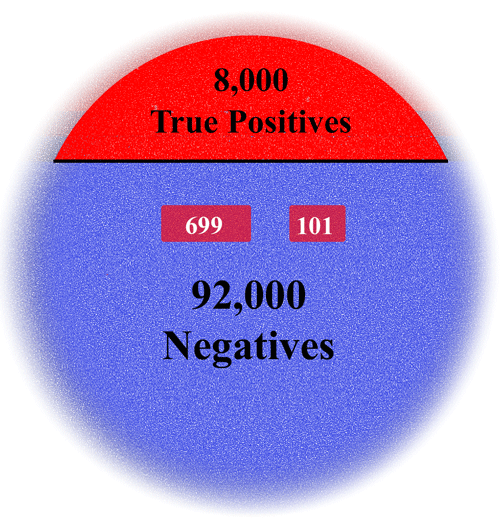The calculation of the lowest end of the recall range is based on the high end of the False Negatives projection: Rl = TP / (TP+FNh) = 8,000 / (8,000 + 699) = 91.96%

The calculation of the highest end of the recall range is based on the low end of the False Negatives projection: Rh = TP / (TP+FNl) = 8,000 / (8,000 + 101) = 98.75%.

Our final recall range values for this first hypothetical is thus from 92%- 99% recall. It was an unusually good result.

It is important to note that we could have still failed this quality assurance test, in spite of the high recall range shown, if any of the five False Negatives found was a highly relevant, or unique-strong relevant document. That is in accord with the accept on zero error standard that I always apply to the final elusion sample, a standard having nothing directly to do with ei-Recall. Still, I recommend that the e-discovery community also accept this as a corollary to implement ei-Recall. I have previously explained this zero error quality assurance protocol on this blog several times, most recently in Visualizing Data in a Predictive Coding Project – Part Three where I explained:

I always use what is called an accept on zero error protocol for the elusion test when it comes to highly relevant documents. If any are highly relevant, then the quality assurance test automatically fails. In that case you must go back and search for more documents like the one that eluded you and must train the system some more. I have only had that happen once, and it was easy to see from the document found why it happened. It was a black swan type document. It used odd language. It qualified as a highly relevant under the rules we had developed, but just barely, and it was cumulative. Still, we tried to find more like it and ran another round of training. No more were found, but still we did a third sample of the null set just to be sure. The second time it passed.

Variations of First Example with Higher False Negatives Ranges

I want to provide two variations of this hypothetical where the sample of the null set, Negatives, finds more mistakes, more False Negatives. Variations like this will provide a better idea of the impact of the False Negatives range on the recall calculations. Further, the first example wherein I assumed that only five mistakes were found in a sample of 1,534 is somewhat unusual. A point projection ratio of 0.33% for elusion is on the low side for a typical legal search project. In my experience in most projects a higher rate of False Negatives will be found, say in the 0.5% to 2% range.

Let us assume for the first variation that instead of finding 5 False Negatives, we find 20. That is a quadrupling of the False Negatives. It means that we found 1,514 True Negatives and 20 False Negatives in the sample of 1,534 documents from the 92,000 document discard pile. This creates a point projection of 1.30% (20 / 1534), and a binomial range of 0.8% to 2.01%. This generates a projected range of total False Negatives of from 736 (92,000 * .8%) to 1,849 (92,000 * 2.01%).

Now let’s see how this quadrupling of errors found in the sample impacts the recall range calculation.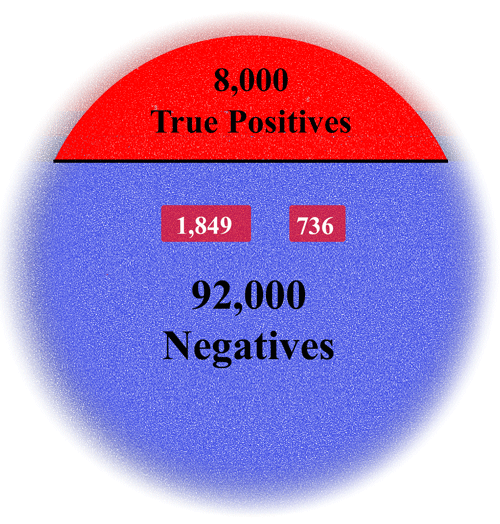The calculation of the low end of the recall range is based on the high end of the False Negatives projection: Rl = TP / (TP+FNh) = 8,000 / (8,000 + 1,849) = 81.23%

The calculation of the high end of the recall range is based on the low end of the False Negatives projection: Rh = TP / (TP+FNl) = 8,000 / (8,000 + 736) = 91.58%.

Our final recall range values for this variation of the first hypothetical is thus 81% – 92%.

In this first variation the quadrupling of the number of False Negatives found at the end of the project, from 5 to 20, caused an approximate 10% decrease in recall values from the first hypothetical where we attained a recall range of 92% to 99%.

Let us assume a second variation that instead of finding 5 False Negatives, finds 40. That is eight times the number of False Negatives found in the first hypothetical. It means that we found 1,494 True Negatives and 40 False Negatives in the sample of 1,534 documents from the 92,000 document discard pile. This creates a point projection of 2.61% (40/1534), and a binomial range of 1.87% to 3.53%. This generates a projected range of total False Negatives of from 1,720 (92,000*1.87%) to 3,248 (92,000*3.53%).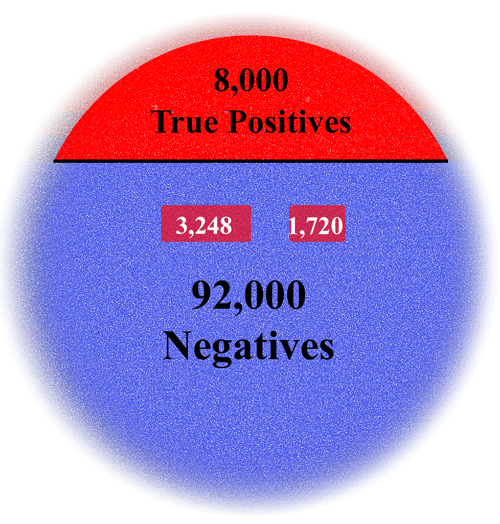The calculation of the low end of the recall range is based on the high end of the False Negatives projection: Rl2 = TP / TP+FNh = 8,000 / (8,000 + 3,248) = 71.12%

The calculation of the high end of the recall range is based on the low end of the False Negatives projection: Rh2 = TP / TP+FNl = 8,000 / (8,000 + 1,720) = 82.30%.

Our recall range values for this variation of the first hypothetical is thus 71% – 82%.

In this second variation the eightfold increase of the number of False Negatives found at the end of the project, from 5 to 20, caused an approximate 20% decrease in recall values from the first hypothetical where we attained a recall range of 92% to 99%.

Second Example of How to Calculate Recall Using the ei-Recall Method

We will again go back to the second example used in In Legal Search Exact Recall Can Never Be KnownThe second hypothetical assumes a total collection of 1,000,000 documents and that 210,000 relevant documents were found and verified.

In the random sample of 1,534 documents (95%+/-2.5%) from the 790,000 documents withheld as irrelevant (1,000,000 – 210,000) we assume that only ten mistakes were uncovered, in other words, 10 False Negatives. Conversely, we found that 1,524 of the documents picked at random from the discard pile (another name for the Negatives) were in fact irrelevant as expected; they were True Negatives.

The percentage of False Negatives in this sample was thus 0.65% (10/1534). Using the binomial interval calculation the range would be from 0.31% to 1.2%. The range generated by the binomial interval is from  2,449 (790,000*0.31%) to 9,480 (790,000*1.2%) False Negatives.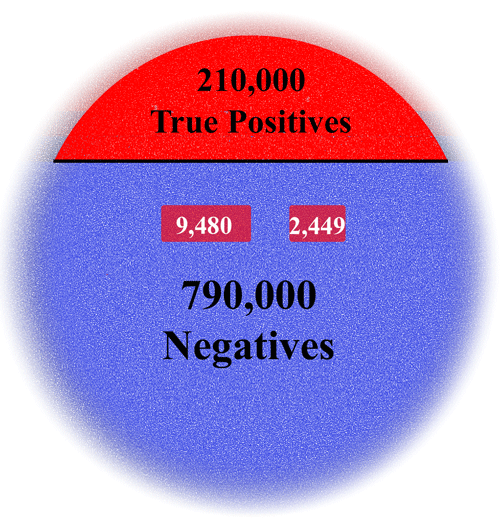The calculation of the lowest end of the recall range is based on the high end of the False Negatives projection: Rl2 = TP / TP+FNh = 210,000 / (210,000 + 9,480) = 95.68%

The calculation of the highest end of the recall range is based on the low end of the False Negatives projection: Rh2 = TP / TP+FNl = 210,000 / (210,000 + 2,449) = 98.85%.

Our recall range for this second hypothetical is thus 96% – 99% recall. This is a highly unusual, truly outstanding result. It is, of course, still subject to the outlier result uncertainty inherent in the confidence level. In that sense my labels on the diagram below of “worst” or “best” case scenario are not correct. It could be better or worse in five times out of one hundred times the sample is drawn in accord with the 95% confidence level. See the discussion near the end of my article In Legal Search Exact Recall Can Never Be Known, regarding the role that luck necessarily plays in any random sample. This could have been a lucky draw, but nevertheless, it is just one quality assurance factor among many, and is still an extremely good recall range achievement.

Variations of Second Example with Higher False Negatives Ranges

I now offer three variations of the second hypothetical where each has a higher False Negative rate. These examples should better illustrate the impact of the elusion sample on the overall recall calculation.

Let us first assume that instead of finding 10 False Negatives, we find 20, a doubling of the rate. This means that we found 1,514 True Negatives and 20 False Negatives in the sample of 1,534 documents in the 790,000 document discard pile. This creates a point projection of 1.30% (20/1534), and a binomial range of 0.8% to 2.01%. This generates a projected range of total False Negatives of from 6,320 (790,000*.8%) to 15,879 (790,000*2.01%).Now let us see how this doubling of errors in the second sample impacts the recall range calculation.

The calculation of the low end of the recall range is: Rl = TP / (TP+FNh) = 210,000 / (210,000 + 15,879) = 92.97%

The calculation of the high end of the recall range is: Rh = TP / (TP+FNl) = 210,000 / (210,000 + 6,320) = 97.08%.

Our recall range for this first variation of the second hypothetical is thus 93% – 97%

The doubling of the number of False Negatives from 10 to 20, caused an approximate 2.5% decrease in recall values from the second hypothetical where we attained a recall range of 96% to 99%.

Let us assume a second variation where instead of finding 10 False Negatives at the end of the project, we find 40. That is a quadrupling of the number of False Negatives found in the first hypothetical. It means that we found 1,494 True Negatives and 40 False Negatives in the sample of 1,534 documents from the 790,000 document discard pile. This creates a point projection of 2.61% (40/1534), and a binomial range of 1.87% to 3.53%. This generates a projected range of total False Negatives of from 14,773 (790,000*1.87%) to 27,887 (790,000*3.53%).The calculation of the low end of the recall range is now: Rl = TP / (TP+FNh) = 210,000 / (210,000 + 27,887) = 88.28%

The calculation of the high end of the recall range is now: Rh = TP / (TP+FNl) = 210,000 / (210,000 + 14,773) = 93.43%.

Our recall range for this second variation of second hypothetical is thus 88% – 93%.

The quadrupling of the number of False Negatives from 10 to 40, caused an approximate 7% decrease in recall values from the original where we attained a recall range of 96% to 99%.

If we do a third variation and increase the number of False Positives found by eight-times, from 10 to 80, this changes the point projection to 5.22% (80/1534), with a binomial range of 4.16% to 6.45%.  This generates a projected range of total False Negatives of from 32,864 (790,000*4.16%) to 50,955 (790,000*6.45%).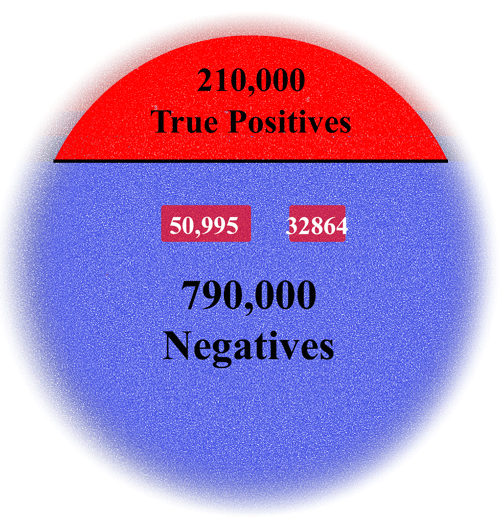The calculation of the low end of the recall range is: Rl = TP / (TP+FNh) = 210,000 / (210,000 + 50,955) = 80.47%.

The calculation of the high end of the recall range is: Rh = TP / (TP+FNl) = 210,000 / (210,000 + 32,864) = 86.47%.

Our recall range for this third variation of the second hypothetical is thus 80% – 86%.

The eightfold increase of the number of False Negatives, from 10 to 80, caused an approximate 15% decrease in recall values from the second hypothetical where we attained a recall range of 96% to 99%.

By now you should have a pretty good idea of how the ei-Recall calculation works, and a feel for how the number of False Negatives found impacts the overall recall range.

Third Example of How to Calculate Recall Using the ei-Recall Method where there is Very Low Prevalence

A criticism of many recall calculation methods is that they fail and become completely useless in very low prevalence situations, say 1%, or sometimes even less. Such low prevalence is considered by many to be common in legal search projects.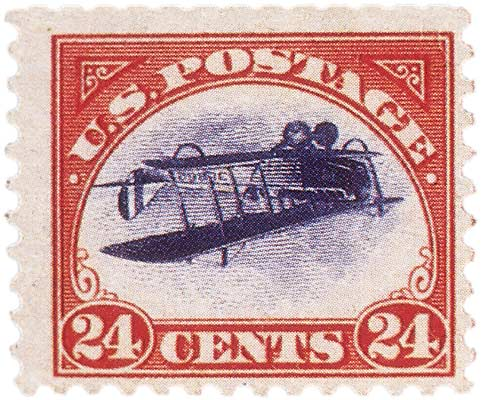Obviously it is much harder to find things that are very rare, such as the famous, and very valuable, Inverted Jenny postage stamp with the upside down plane. These stamps exist, but not many. Still, it is at least possible to find them (or buy them), as opposed to a search for a Unicorn or other complete fiction. (Please, Unicorn lovers, no hate mail!) These creatures cannot be found no matter how many searches and samples you take because they do not exist. There is absolute zero prevalence.This circumstance sometimes happens in legal search, where one side claims that mythical documents must exist because they want them to. They have a strong suspicion of their existence, but no proof. More like hope, or wishful thinking. No matter how hard you look for such smoking guns, you cannot find them. You cannot find something that does not exist. All you can do is show that you made reasonable, good faith efforts to find the Unicorn documents, and they did not appear. Recall calculations make no sense in crazy situations like that because there is nothing to recall. Fortunately that does not happen too often, but it does happen, especially in the wonderful world of employment litigation.

We are not going to talk further about a search for something that does not exist, like a Unicorn, the zero prevalence. We will not even talk about the extremely, extremely rare, like the Inverted Jenny. Instead we are going to talk about prevalence of about 1%, which is still very low.

In many cases, but not all, very low prevalence like 1%, or less, can be avoided, or at least mitigated, by intelligent culling. This certainly does not mean filtering out all documents that do not have certain keywords. There are other, more reliable methods than simple keywords to eliminate superfluous irrelevant documents, including elimination by file type, date ranges, custodians, and email domains, among other things.

When there is a very low prevalence of relevant documents, this necessarily means that there will be a very large Negatives pool, thus diluting the sampling. There are ways to address the large Negatives sample pool, as I discussed in Part One. The most promising method is to cull out the low end of the probability rankings where relevant documents should anyway be non-existent.

Even with the smartest culling possible, low prevalence is often still a problem in legal search. For that reason, and because it is the hardest test for any recall calculation method, I will end this series of examples with a completely new hypothetical that considers a very low prevalence situation of only 1%. This means that there will be a large size Negatives pool: 99% of the total collection.

We will again assume a 1,000,000 document collection, and again assume sample sizes using 95% +/-2.5% confidence level and interval parameters. An initial sample of all documents taken at the beginning of the project to give us a rough sense of prevalence for search guidance purposes (not recall calculations), projected a range of relevant documents of from 5,500 to 16,100.

The lawyers in this hypothetical legal search project plodded away for a couple of weeks and found and confirmed 9,000 relevant documents, True Positives all. At this point they are finding it very difficult and time consuming to find more relevant documents. What they do find is just more of the same. They are sophisticated lawyers who read my blog and have a good grasp of the nuances of sampling. So they know better than to simply rely on a point projection of prevalence to calculate recall, especially one based on a relatively small sample of a million documents taken at the beginning of the project. See In Legal Search Exact Recall Can Never Be KnownThey know that their recall level could be only a 56% recall 9,000/16,100 (or perhaps far less, in the event the one sample they took was a confidence level outlier event, or there was more concept drift than they thought). It could also be near perfect, 100% recall, when they consider the binomial interval range going the other way. The 9,000 documents they had found was way more than the low range of 5,500. But they did not really consider that too likely.

They decide to stop the search and take a second 1,534 document sample, but this time of the 991,000 null set (1,000,000 – 9,000). They want to follow the ei-Recall method, and they also want to test for any highly relevant or unique strong relevant documents by following the accept on zero error quality assurance test. They find -1- relevant document in that sample. It is just a more of the same type merely relevant document. They had seen many like it before. Finding a document like that meant that they passed the quality assurance test they had set up for themselves. It also meant that using the binomial intervals for 1/1534, which is from 0.00% and 0.36%, there is a projected range of False Negatives of from between -0- and 3,568 documents (991,000*0.36%). (Actually, a binomial calculator that shows more decimal places than any I have found on the web (hopefully we can fix that soon) will not show zero percent, but some very small percentage less than one hundredth of a percent, and thus some documents, not -0- documents, and thus something slightly less than 100% recall.)They then took out the ei-Recall formula and plugged in the values to see what recall range they ended up with. They were hoping it was tighter, and more reliable, than the 56% to 100% recall level they calculated from the first sample alone based on prevalence.

Calculation for the low end of the recall range: Rl = TP / (TP+FNh) = 9,000 / (9,000 + 3,568) = 71.61%.

Calculation for the high end of the recall range: Rh = TP / (TP+FNl) = 9,000 / (9,000 + 0) = 100%.

The recall range using ei-Recall was 72% – 100%.

The attorneys’ hopes in this extremely low prevalence hypothetical were met. The 72%-100% estimated recall range was much tighter than the original 56%-100%. It was also more reliable because it was based on a sample taken at the end of the project when relevance was well defined. Although this sample did not, of and by itself, prove that a reasonable legal effort had been made, it did strongly support that position. When considering all of the many other quality control efforts they could report, if challenged, they were comfortable with the results. Assuming that they did not miss a highly relevant document that later turns up in discovery, it is very unlikely they will ever have to redo, or even continue, this particular legal search and review project.

Would the result have been much different if they had doubled the sample size, and thus doubled the cost of this quality control effort? Let us do the math and find out, assuming that everything else was the same.This time the sample is 3,068 documents from the 991,000 null set. They find two relevant documents, False Negatives, of a kind they had seen many times before. This created a binomial range of 0.01%  to 0.24%, projecting a range of False Negatives from 99 to 2,378 (991,000 * 0.01% — 991,000 * 0.24%). That creates a recall range of 79% – 99%.

Rl = TP / (TP+FNh) = 9,000 / (9,000 + 2,378) = 79.1%.

Rh = TP / (TP+FNl) = 9,000 / (9,000 + 99) = 98.91%.

In this situation by doubling the sample size the attorneys were able to narrow the recall range from 72% – 100% to 79% – 99%. But was it worth the effort and doubling of  cost? I do not think so, at least not in most cases. But perhaps in larger cases, it would be worth the expense to tighten the range somewhat and so increase somewhat the defensibility of your efforts. After all, we are assuming in this hypothetical that the same proportional results would turn up in a sample size double that of the original. The results could have been much worse, or much better. Either way, your results would be more reliable than an estimate based on a sample half that size, and would have produced a tighter range. Also, you may sometimes want to take a second sample of the same size, if you suspect the first was an outlier.

Let is consider one more example, this time of an even smaller prevalence and larger document collection. This is the hardest challenge of all, a near Inverted Jenny puzzler. Assume a document collection of 2,000,000 and a prevalence based on a first random sample for search-help purposes, where again only one relevant was found in the sample of 1,534 sample. This suggested there could be as many as 7,200 relevant documents (0.36% * 2,000,000). So in this second hypothetical we are talking about a dataset where the prevalence may be far less than one percent.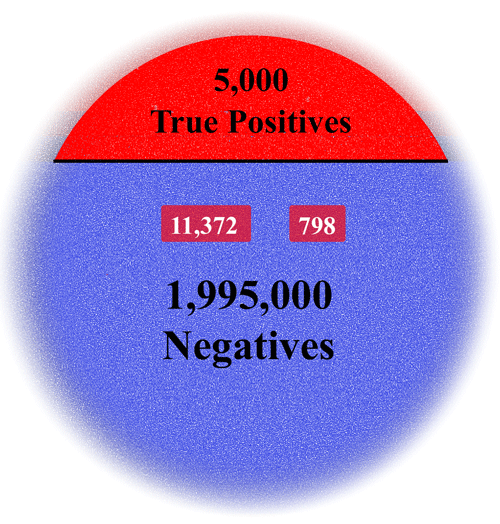Assume next that only 5,000 relevant documents were found, True Positives. A sample 1,534 of the remaining 1,995,000 documents found -3- relevant, False Negatives. The binomial intervals for 3/1534, is from 0.04% to 0.57%, producing  a projected range of False Negatives of from between 798 and 11,372 documents (1,995,000 * .04% — 1,995,000 * 0.57%). Under ei-Recall the recall range measured is 31% – 86%.

Rl = TP / (TP+FNh) = 5,000 / (5,000 + 11,372) = 30.54%.

Rh = TP / (TP+FNl) = 5,000 / (5,000 + 798) = 86.24%.

31% – 86% is a big range. Most would think too big, but remember, it is just one quality assurance indicator among many.

The size of the range could be narrowed by a larger sample. (It is also possible to take two samples, and, with some adjustment, add them together as one sample. This is not mathematically perfect, but fairly close, if you adjust for any overlaps, which anyway would be unlikely.) Assume the same proportions where we sample 3,068 documents from 1,995,000 Negatives, and find -6- relevant, False Negatives. The binomial range is 0.07% – 0.43%. The projected number of False Negatives is 1,397 – 8,579 (1,995,000*.07% – 1,995,000*.43%). Under ei-Recall the range is 37% – 78%.

Rl = TP / (TP+FNh) = 5,000 / (5,000 + 8,579) = 36.82%.

Rh = TP / (TP+FNl) = 5,000 / (5,000 + 1,397) = 78.16%.

The range has been narrowed, but is still very large. In situations like this, where there is a very large Negatives set, I would suggest taking a different approach. As discussed in Part One, you may want to consider a rational culling down of the Negatives. The idea is similar to that behind stratified sampling. You create a subset or strata of the entire collection of Negatives that has a higher, hopefully much higher prevalence of False Negatives than the entire set. See eg. William Webber, Control samples in e-discovery (2013) at pg. 3Although Webber’s paper only uses keywords as an example of an easy way to create a strata, in reality in modern legal search today there are a number of methods that could be used to create the stratas, only one of which is keywords. I use a combination of many methods that varies in accordance with the data set and other factors. I call that a multimodal method. In most cases (but not all), this is not too hard to do, even if you are doing the stratification before active machine learning begins. The non-AI based culling methods that I use, typically before active machine learning begins, include parametric Boolean keywords, concept, key player, key time, similarity, file type, file size, domains, etc.

After the predictive coding begins and ranking matures, you can also use probable relevance ranking as a method of dividing documents into strata. It is actually the most powerful of the culling methods, especially when it comes to predicting irrelevant documents. The second filter level is performed at or near the end of a search and review project. (This is all shown in the two-filter diagram above, which I may explain in greater detail in a future blog.) The second AI based filter can be especially effective in limiting the Negatives size for the ei-Recall quality assurance test. The last example will show how this works in practice.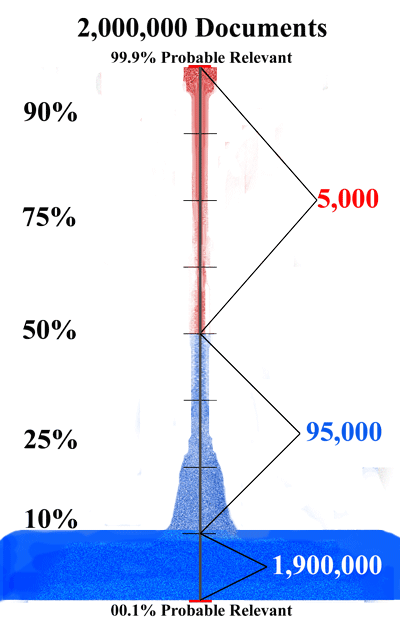We will begin this example as before, assuming again 2,000,000 documents where the search finds only 5,000. But this time before we take a sample of the Negatives we divide them into two strata. Assume, as we did in the example we considered in Part One, that the predictive coding resulted in a well defined distribution of ranked documents. Assume that all 5,000 documents found were in the 50%, or higher, probable relevance ranking (shown in red in the diagram). Assume that all of the 1,995,000 presumed irrelevant documents are ranked 49.9%, or less, probable relevant (shown in blue in the diagram). Finally assume that 1,900,000 of these documents are ranked 10% or less probable relevant. Thus leaving 95,000 documents ranked between 10.1% and 49.9%.

Assume also that we have good reason to believe based on our experience with the software tool used, and the document collection itself, that all, or almost all, False Negatives are contained in the 95,000 group. We therefore limit our random sample of 1,534 documents to the 95,000 lower midsection of the Negatives. Finally, assume we now find -30- relevant, False Negatives, none of them important.The binomial range is 0.80% – 2.01%, but this time the projected number of False Negatives is 1,254 – 2,641 (95,000*1.32%  — 95,000*2.78%). Under ei-Recall the range is 72.37% – 80.06%.

Rl = TP / (TP+FNh) = 5,000 / (5,000 + 2,641) = 72.37%.

Rh = TP / (TP+FNl) = 5,000 / (5,000 + 1,245) = 80.06%.

We see that culling down the Negative set of documents in a defensible manner can lead to a much tighter recall range. Assuming we did the culling correctly, the resulting recall range would also be more accurate. On the other hand, if the culling was wrong, based on incorrect presumptions, then the resulting recall range would be less accurate.

The fact is, no random sampling techniques can provide completely reliable results in very low prevalence data sets. There is no free lunch, but, at least with ei-Recall the bill for your lunch is honest because it includes ranges. Moreover, with intelligent culling to increase the probable prevalence of False Negatives, you are more likely to get a good meal.

Conclusion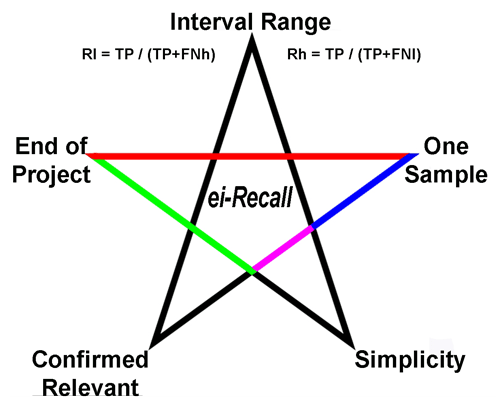There are five basic advantages of ei-Recall over other recall calculation techniques:

1. Interval Range values are calculated, not just a deceptive point value. As shown by In Legal Search Exact Recall Can Never Be Known, recall statements must include confidence interval range values to be meaningful.
2. One Sample only is used, not two, or more. This limits the uncertainties inherent in multiple random samples.
3. End of Project is when the sample of the Negatives is taken for the calculation. At that time the relevance scope has been fully developed.
4. Confirmed Relevant documents that have been verified as relevant by iterative reviews, machine and human, are used for the True Positives. This eliminates another variable in the calculation.
5. Simplicity is maintained in the formula by reliance on basic fractions and common binomial confidence interval calculators. You do not need an expert to use it.

I suggest you try ei-Recall. It has been checked out by multiple information scientists and will no doubt be subject to more peer review here and elsewhere. Be cautious in evaluating any criticisms you may read of ei-Recall from persons with a vested monetary interest in the defense of a competitive formula, especially vendors, or experts hired by vendors. Their views may be colored by their monetary interests. I have no skin in the game. I offer no products that include this method. My only goal is to provide a better method to validate large legal search projects, and so, in some small way, to improve the quality of our system of justice. The law has given me much over the years. This method, and my other writings, are my personal payback.

I offer ei-Recall to anyone and everyone, no strings attached, no payments required. Vendors, you are encouraged to include it in your future product offerings. I do not want royalties, nor even insist on credit (although you can do so if you wish, assuming you do not make it seem like I endorse your product). ei-Recall is all part of the public domain now. I have no product to sell here, nor do I want one. Although I do hope to create an online calculator soon for ei-Recall. When I do, that too will be a give away.My time and services as a lawyer to implement ei-Recall are not required. Simplicity is one of its strengths, although it helps if you are part of the eLeet. I think I have fully explained how it works in this lengthy article. Still, if you have any non-legal technical questions about its application, send me an email, and I will try to help you out. Gratis of course. Just realize that I cannot by law provide you with any legal advice. All articles in my blog, including this one, are purely for educational services, and are not legal advice, nor in any way a solicitation for legal services. Show this article to your own lawyer or e-discovery vendor. You do not have to be 1337 to figure it out (although it helps).

### 4 Responses to Introducing “ei-Recall” – A New Gold Standard for Recall Calculations in Legal Search – Part Three

1.Bill Dimm says:

“The most promising method is to cull out the low end of the probability rankings where relevant documents should anyway be non-existent.”

I would not recommend doing that. The optimum number of samples to pull from a stratum is known to be (approximately) proportional to N*sqrt(p*(1-p)), where N is the number of documents in the stratum and p is the probability of a document in the stratum being relevant. Of course, you don’t know p, but if your stratification is generated from relevance scores (not something like identifying email senders that are external newsletters, which are known with certainty to be non-relevant) it is certainly not valid to assume that p is zero. You are actually committing two errors by ignoring the stratum — you are biasing the recall estimate to be too high (you are undercounting false negatives by assuming there are absolutely none in the stratum you are discarding) and you are underestimating how wide your confidence interval should be by ignoring the uncertainty in the number of false negatives in that stratum. So, your computed confidence interval is too narrow and it is centered at a value that is too high.

For your example, if you sampled 3,000 documents from your 1,900,000 document stratum and found NONE of them to be relevant, the confidence interval for the prevalence for that stratum would be 0% to 0.1227%, giving a range of 0 to 2331 false negatives for that stratum. Notice that even with a point estimate of 0, and a bigger sample, the upper bound for that stratum is as big as your upper bound for the 95,000 document stratum. There is a ton of uncertainty from that stratum that is not reflected in your confidence interval.

“Actually, a binomial calculator that shows more decimal places than any I have found on the web (hopefully we can fix that soon)”

“See eg. William Webber, Control samples in e-discovery (2013) at pg. 3”

Is there a link to this paper available? I can’t seem to find any mention of the paper anywhere else (even in William’s CV).

In your second calculation, you have:
8,000 / (8,000 + 736) = 86.99%
It should be 91.58%

Finally, it is worth noting that the number of false negatives you use in some of your samples implies a rather high point estimate for the recall. For a given sample size, parameters that give a higher point estimate are also going to give a narrower confidence interval. People who are aiming for more modest recall should be warned not to expect their confidence intervals to be as narrow with a 1,534 document sample.

For example, your example with 9,000 true positives and 991,000 negatives involves a sample of 1,534 documents where only 1 is a false negative, giving a recall point estimate of 93.3%. In that case, having 1 relevant document in a sample of 1,534 would be a fairly likely event if your actual (but unknown) recall was 93.3%. Your calculation gives a recall range of 72% to 100%, so the lower bound isn’t bad, but that’s largely because your assumptions are consistent with recall that is rather high. If the actual (but unknown) recall were 78%, instead, it is possible that you would find 1 false negative in a sample of 1,534, but not terribly likely. It would be much more likely that you would find 4, which would lead to a recall estimate of 58% to 93% (the interval is both wider and centered at a lower value). That lower bound, while not terrible, is low enough that it may cause some concern. And, there is a roughly 50% chance that you would (due to randomness) find more than 4 relevant documents in the sample, leading to an even lower lower bound. So, if you are normally getting around 93% recall your example might be typical of your experience, but if you normally get recall more in the 75-80% range you should not expect to get a lower bound like 72% from a sample of 1,534 documents — expect something more in the 50-60% range for the lower bound.

2.Ralph Losey says:

I especially appreciate your catching my simple division error in one of my 12 examples. I have since corrected it. I checked the math so many times and still made a stupid mistake! Remarkable. It was a small error, but I always want to be accurate. I hope you will proof read all of my math, assuming I ever do a blog like this again. Much appreciated.

Your calculator that you refer to, could you possible add another entry field designed for “binomial,” and not requiring the “1000 times larger” hack your calculator suggests the user do to create a binomial result?

I did not know William had not published this paper until after I published this blog. But he has since given me permission to share it. So please email me and I will reply with a copy, or you could ask him direct.

Re you comments that the interval increases as more False Negatives are found, yes, that is to be expected. The lower the recall the higher the range.

On your caution about using strata of the negatives. I agree with your general caution, and said so in my blog. But I appear to have a much more optimistic view than you do that in some projects you can be reasonably certain based on “heuristic” factors that the lower strata is clear, or very close to clear of False Negatives.

When it comes to sampling one can never know anything for sure. It is a question of reasonable estimates. As a lawyer I am used to that. There is rarely any certainty in the law. It is all about reasonability, not perfection, especially when it comes to Rule 26(g).

I think I said a couple of times in this blog series that there are always uncertainties with sampling. Even in my book ei-Recall is just one of many factors to consider in quality assurance of the reasonableness of the efforts. There are quite a few others, including many that are experience based, not not metric.

Probably the thing that I found most encouraging from your comment, is what you did *not* say. You did not find anything terribly wrong, other the one small math error, and you did not express disagreement as to the overall value of ei-Recall, just the weaknesses inherent in any sampling, nor, and this is critical, you did not suggest any another method that you think is better.

3.Bill Dimm says:

“Your calculator that you refer to, could you possible add another entry field designed for ‘binomial,’ and not requiring the ‘1000 times larger’ hack your calculator suggests the user do to create a binomial result?”

Can you explain why you want this? The correct probability distribution for sampling in the way it is normally done in e-discovery (i.e., without replacement) is the hypergeometric distribution, which is what my calculator uses. As far as I know, the only reason the binomial distribution is used in e-discovery is as an approximation to the hypergeometric distribution that is easier to calculate. Granted, the binomial distribution is a very good approximation to the hypergeometric distribution in almost all situations that you are likely to encounter in e-discovery (one possible exception being stratified sampling where some strata are rather small), but why use an approximate result when the exact result is already available to you without any need to do the hard math (since I already did it for you when I built the calculator)?

4. […] this method, and the diagram shown here illustrating it, at the conclusion of my blog series, Introducing “ei-Recall” – A New Gold Standard for Recall Calculations in Legal Search – Part…. I use the two-filter method in most large projects as part of my overall multimodal, bottom line […]